## Bicentric Polygon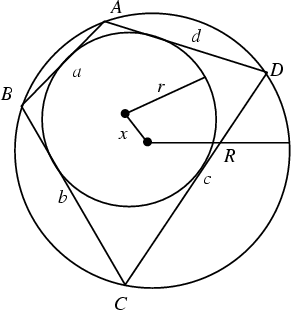A Polygon which has both a Circumcircle (which touches each vertex) and an Incircle (which is tangent to each side). All Triangles are bicentric with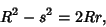(1)

whereis the Circumradius,is the Inradius, andis the separation of centers. In 1798, N. Fuss characterized bicentric Polygons of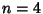, 5, 6, 7, and 8 sides. For bicentric Quadrilaterals (Fuss's Problem), the Circles satisfy(2)

(Dörrie 1965) and(3)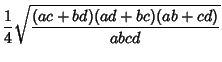(4)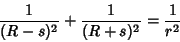(5)

and(6)

The Area of a bicentric quadrilateral is(7)

If the circles permit successive tangents around the Incircle which close the Polygon for one starting point on the Circumcircle, then they do so for all points on the Circumcircle.

Dörrie, H. Fuss' Problem of the Chord-Tangent Quadrilateral.'' §39 in 100 Great Problems of Elementary Mathematics: Their History and Solutions. New York: Dover, pp. 188-193, 1965.